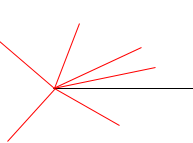SEARCH HOMEMath Central Quandaries & QueriesQuestion from abhi: how to calculate the angle between two lines, given the length of the lines.. angle should vary from 0 - 360 in the counterclockwise directionAbhi,

If you have two lines of fixed lengths then you can put them together with any angle between them. In my diagram one of the lines is the black line and the other is the red line which I can use to make any angle I like between them.Do you have a triangle where you know the lengths of the three sides and you want to find the angles? If so you can use the Law of Cosines.

Harley

You cannot do this; the information is not sufficient. You can have (say) a line of length 3 and a line of length 4 making any angle that you choose. Imagine two sticks hinged together at one end.

If you know all three sides of a triangle you can use the cosine law from trigonometry:

a² = b² + c² -2bc cos(α)

where α is the angle between the sides of length b and c.

First solve for cos(α) [which may be positive or negative] and then for α itself. The angle will be somewhere from 0 to 180 degrees.

-RDMath Central is supported by the University of Regina and The Pacific Institute for the Mathematical Sciences.